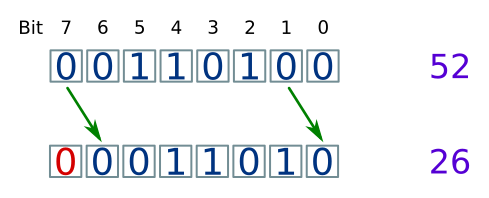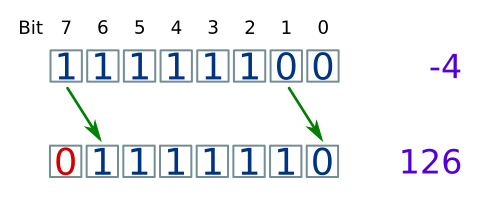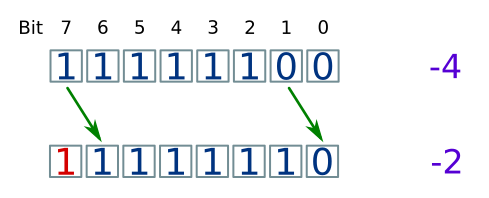# Binary shift

Martin McBride, 2017-02-24
Tags binary shift logical shift arithmetic shift multiply divide
Categories data representation numbers

Binary shifting is a simple but useful method of bit manipulation, often used alongside bitwise logical operations.

A normal bit shift operation is sometimes called a logical shift, because it treats the byte as a set of independent logical bits. The alternative is an arithmetic shift, which treats the byte as a number.

{{% yellow-note%}} The examples here all use bytes. You can also apply the same technique to 16-bit words, 32-bit words or any other word size. {{% /yellow-note%}}

## Logical shift left

The shift left operation shifts each bit one place to the left:• What was in bit position 0 moves to bit position 1.
• What was in bit position 1 moves to bit position 2.
• etc...
• What was in bit position 7 (the left most bit) falls off the end and is lost forever.
• A 0 is moved into bit position 0.

You will notice in the example, the byte originally had a denary value 29. After the shift, it has a denary value 58, which is exactly twice as much as its original value. That will always be the case.

It shouldn't be a surprise, because a similar thing happens in base 10. If we take the number 237 and shift each digit 1 place to the left, adding a zero to the right hand side, we get 2370. This is, of course, 10 times the starting value - that is how you multiply things by 10. In binary, left shifting multiplies by 2, not 10, because we are working in base 2.

What happens if we shift left by 3 bits? Take a look:Each bit is shifted 3 places to the left. The 3 bits at the right of the byte are pushed off the end, and are lost. Three 0 bits are placed in bit positions 0, 1, 2.

{{% green-note %}} Shifting by 3 places is exactly the same as shifting by 1 place, 3 times! {{% /green-note %}}

Looking at the denary value, the initial byte has a value of 14. After shifting by 3, the new value is 112 (14x8). As you might expect, shifting left by 3 is equivalent to multiplying by 2x2x2 (three 1 bit shifts).

{{% orange-note %}} Shifting left by n bits is equivalent to multiplying by 2 to the power n. {{% /orange-note %}}

## Logical shift right

The shift right operation shifts each bit one place to the left:• What was in bit position 0 (the right most bit) falls off the end and is lost forever.
• What was in bit position 1 moves to bit position 0.
• What was in bit position 2 moves to bit position 1.
• etc...
• A 0 is moved into bit position 7.

In the example, the byte originally had a denary value 52. After the shift, it has a denary value 26, which is half of the original value. exactly twice as much as its original value. That will always be the case.

Once again, a similar thing happens in base 10. If we take the number 350 and shift each digit 1 place to the right, we get 35. This is, of course, a tenth of starting value. In binary, right shifting divides by 2, not 10, because we are working in base 2.

What happens if the original number doesn't dived exactly? Here is an example. We will shift the binary value 00010110 right by 2:Since this is a 2 bit shift, we would expect the value to be divided by 2 to the power 2 - (2x2), ie 4.

In this case, though, the initial value 22 doesn't divide by 4. The correct result would be 5.5, but we actually get 5.

{{% purple-note %}} When you use right shift to divide a number by a power of 2, the result will be rounded down to an integer value. {{% /purple-note %}}

## Arithmetic shift right

This is a more advanced topic that you mighty not need for GCSE.

If you are dealing with bytes or words which represent signed integers, the logical shift right won't work for negative numbers. For example:The problem here is that bit 7, the sign bit is getting set to 0. This gives an incorrect result - instead of a small negative value, you get a large positive value.

With an arithmetic shift, the sign bit stays the same as the data shifts. If the sign bit was 0, it will be filled with a 0, but if it was 1 it will be filled with a 1:Negative numbers are rounded down, just like positive values. Just to be clear:

• If 3 is right shifted once, it gives 1 (because 1.5 rounded down is 1).
• If -3 is right shifted once, it gives -2 (because -1.5 rounded down is -2).

{{% blue-note %}} You don't really need an arithmetic shift left, because a logical shift left does exactly the same thing. {{% /blue-note %}}